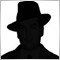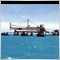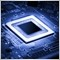• 概述
• 评论 (5)
• 评论 (2)

Bollinger Bands Technical Indicator (BB) is similar to envelopes. The only difference is that the bands of Envelopes are plotted a fixed distance (%) away from the moving average, while the Bollinger Bands are plotted a certain number of standard deviations away from it. Standard deviation is a measure of volatility, therefore Bollinger Bands adjust themselves to the market conditions. When the markets become more volatile, the bands widen and they contract during less volatile periods.

To achieve this, the product of the current Inphase component and its value a bar ago are added to the product of the current Quadrature component and its value one bar ago to produce the real part. For the imaginary part, the product of the current Quadrature component and the value of Inphase component a bar ago is taken away from the product of the current Inphase component and the value of the Quadrature component a bar ago. Both real and imaginary parts are then smoothed, before the cycle period can be calculated.

The cycle is calculated by taking the arctangent of the imaginary part divided by the real part. Restrictions are placed so as to make sure that the rate of change of the cycle period is limited to +-50% of the previous cycle period and further more, the resulting cycle period is limited to being greater than 6 and less than 50. Smoothing is then applied for the last time on the cycle period.

This cycle period value is then used at each bar close as the value that represents the number of bars back to be analyzed for producing the Bollinger bands  indicator. This enables the adaptive nature of the indicator.

### Indicator Parameters:

• The limits value controls the number of bars the indicator will be drawn for .
• Deviation is decimal value that denotes the standard deviation. The lower the number, the narrower the bands of the indicator will be.17225
Nikolay Gaylis 2019.01.14 07:2812010
2018.01.09 19:045430
2017.11.09 14:256086
2017.01.31 07:267168
2016.03.11 06:09